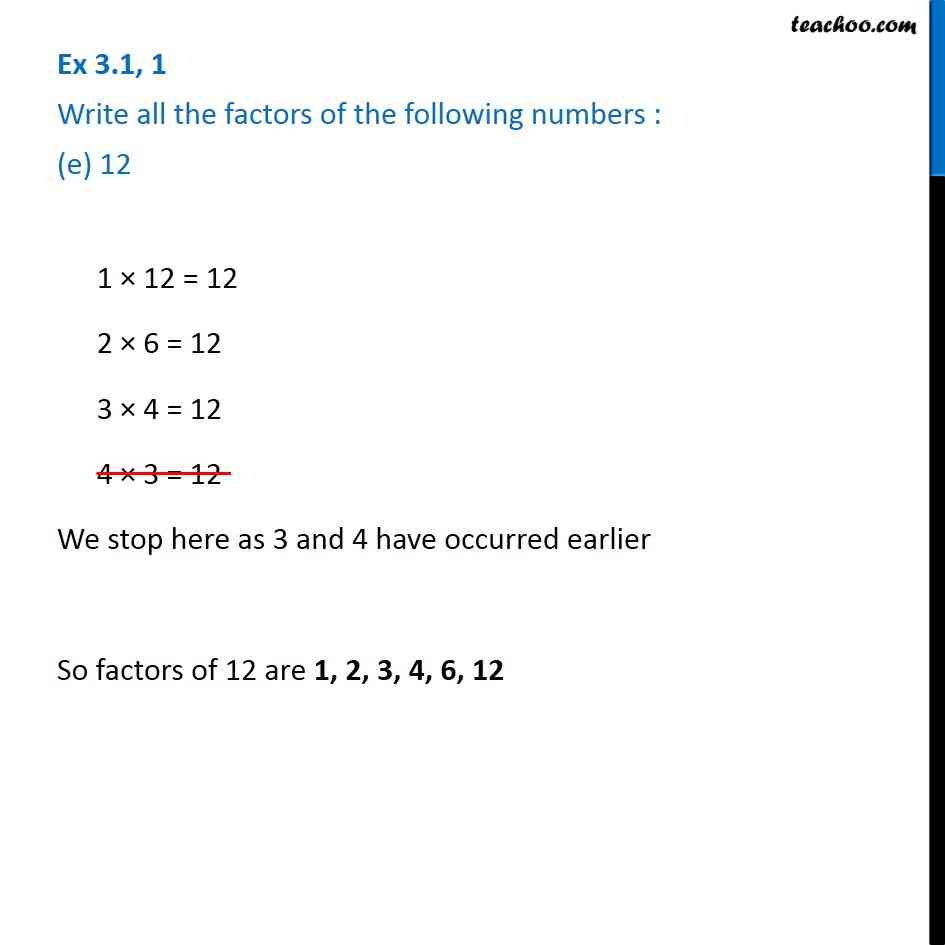1. Chapter 3 Class 6 Playing with Numbers
2. Serial order wise
3. Ex 3.1

Transcript

Ex 3.1, 1 Write all the factors of the following numbers : (e) 12 1 × 12 = 12 2 × 6 = 12 3 × 4 = 12 4 × 3 = 12 We stop here as 3 and 4 have occurred earlier So factors of 12 are 1, 2, 3, 4, 6, 12